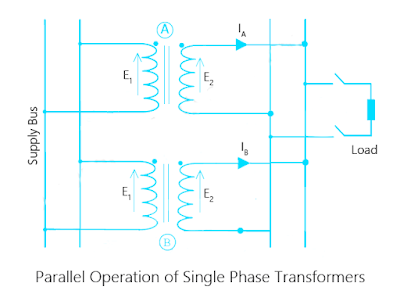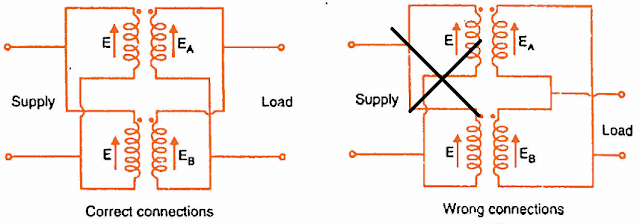# Parallel Operation of Transformers

The transformers are connected in parallel when the load on them is more than the rating of the individual transformers. Before going to discuss parallel operation of single phase transformers  A small question for you all that is ‘why the parallel operation of transformers is required?’. The answer is simple we have some advantages over operating single transformer units so we go for parallel operation of transformers.## Advantages of Parallel Operation of Transformers

Parallel operation is nothing but connecting primary windings of two or more transformers to supply and secondary windings to common loads.To supply more than the rating of existing transformer parallel connections will be employed.It is very economical to operate small no.of transformer units in parallel to supply rated output than a big rated transformer.

#### Parallel operation of transformer because of following advantages

1. Maximum power system efficiency: Power system loads always varies to maintain its efficiency we operate transformers in parallel because transformer gives the maximum efficiency at full load, if we use the single large transformer, the load on the large transformer always varies so it will operate with low efficiency. In another hand, if we operate small units in parallel we can switch on/off as per load demand and we can maintain high efficiency.
2. Ease of electrical power switching: Scheduling the power with the single large transformer will become difficult because of its high rating and distribution system will be connected, controlled by a single transformer we can avoid this problem using parallel operation of transformers.This also improves power system reliability, flexibility.
3. Economical Issues /Non-availability of large transformer: If a large transformer of the required rating is unavailable we can go for small rated transformers which can perform better than the single large unit. And large transformer operating costs will be more than the parallel operation of single phase transformers.
4. Easier transportation: Transportation will be very easy for small transformers: If installation location is far away from transformer manufacturing/selling point.So parallel operation of transformers is to be encouraged.

## Conditions for Parallel Operation of Transformers

We can’t connect any two or more transformers in parallel blindly.When two or more transformers need to operate in parallel, they must meet some conditions for efficient performance.Major conditions for parallel operation of transformers are listed below.
• The voltage ratings and voltage ratios of the transformers should be the same.
• Transformers should be properly connected with regard to their polarities.
• The per unit or percentage impedance of the transformers should be equal.
• The reactance/resistance ratios of the transformers should be the same.

### Same Voltage Rating and Voltage Ratio

The voltage ratios of two transformers must be same but why?
The reason is simple if voltage ratio of two transformers is different and they put in parallel with same primary supply voltage, there will be a difference in secondary voltages. As secondaries of transformers complete a closed loop there will be circulating currents.In this case, the considerable amount of current is drawn by the transformers even without load.As the internal impedance of the transformer is small, a small voltage difference may cause sufficiently high circulating current causing unnecessary extra I²R loss.Circulating Currents=IC= (EA-EB)/ZA+ZB

### Connections with regard to Polarity

The polarity of all connected transformers must be same in order to avoid short circuit.The polarity of a transformer taken w.r.t dot notation.Dots of all transformers connected together on primary and secondary separately. If the polarity is opposite to each other, huge circulating current flows.In the below diagram right and wrong parallel operation connections are shown take a look.### The phase sequence must be identical for all parallel transformers.

This condition is can be applied only to poly-phase transformers. If the phase sequences are not same, then transformers cannot be connected in parallel.During the cycle, each pair of phases will be short-circuited.

### Equal Percentage Impedance

The per unit (PU) impedance of each transformer on its own base must be same.This condition is also desirable for proper parallel operation of single phase transformers. If this condition is not met, the transformers will not share the load according to their kVA ratings.
Sometimes this condition is not fulfilled by the design of the transformers. In that case, it can be corrected by inserting proper amount of resistance or reactance or both in series with either primary or secondary circuits of the transformers where the impedance is below the value required to fulfill this condition.That is why per unit impedance of the connected transformers must be same.